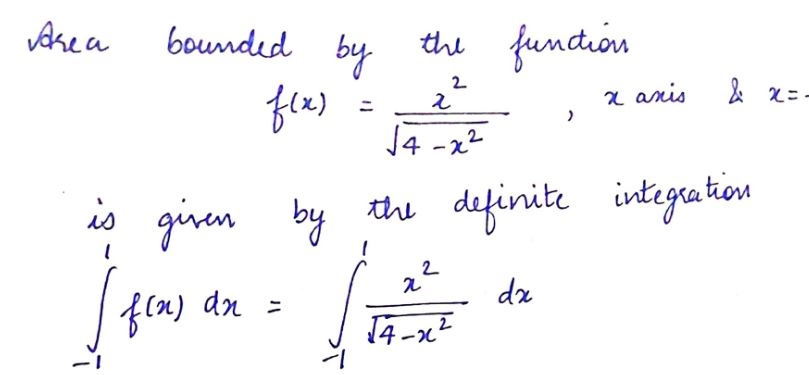# Find the area A of the region R between the graph of f(x)= (x^2)/(4-x^2)^(1/2) and the x-axis on the interval [-1,1].

Question
6 views

Find the area A of the region R between the graph of f(x)= (x^2)/(4-x^2)^(1/2) and the x-axis on the interval [-1,1].

check_circle

Step 1...

### Want to see the full answer?

See Solution

#### Want to see this answer and more?

Solutions are written by subject experts who are available 24/7. Questions are typically answered within 1 hour.*

See Solution
*Response times may vary by subject and question.
Tagged in